### Home > CALC > Chapter 2 > Lesson 2.4.1 > Problem2-142

2-142.
1. Determine the values of the following limits. If the limit does not exist, indicate why not. Homework Help ✎

1.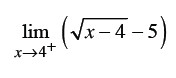2.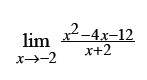3.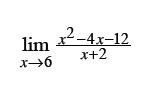4.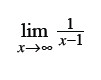5.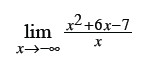6.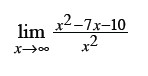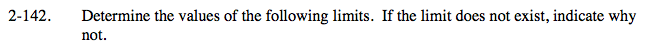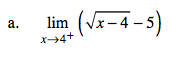This is a one-sided limit because the domain of

$y=\sqrt{x-4}-5 \text{ begins at }x=4.$

Consequently, the limit exists from the right, but not from the left.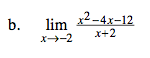Factor first. If you can 'cancel out' the denominator, then the graph has a hole (not a vertical asymptote) and the limit exists.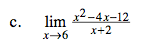Evaluate. The denominator will not equal zero. So the limit and the actual value agree.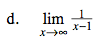$\text{Visualize the graph of }y=\frac{1}{x-1}.$

$\text{It is a transformation of }y=\frac{1}{x}.$

Both graphs have the same end behavior (horizontal asymptote).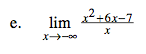For limits in which x→∞ or x→−∞, we are looking for end behavior. For example, is there a horizontal asymptote?

Since the numerator has a higher power, x2, than the denominator, x, there is no horizontal asymptote. Thus the limit goes to either +∞ or −∞.

The limit goes to −∞ because:

$\lim_{x\rightarrow -\infty }\frac{x^{2}}{x}=\lim_{x\rightarrow -\infty }x=-\infty$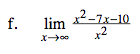Refer to hint in part (e).